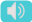ESL Vocabulary about topic 'Math', a small word group with meanings and sentences. The vocabulary has 26 words. The sample words are as follows: calculate, figure, tough time, schoolboy error, ignorant, slow-learner, inherit, impact, stagnate, associate, loathe, add up, .... It helps to enhance speaking and writing skills efficiently in English tests, especially for ESL students who are working for IELTS and TOEFL.
 Free Online Vocabulary TestK12, SAT, GRE, IELTS, TOEFL
 Actions
 Math Vocabulary Word List
calculatev.
compute, evaluate, count
You'll have to calculate the average.
figuren.
the symbol for a number or an amount expressed in numbers
Can you read this figure? Is it a five or an eight?
tough timen.
hard time
Some students have a tough time with math at school due to difficulty reading numbers and following word problems.
schoolboy errorn.
a very basic and stupid mistake
Sending the wrong message to the wrong group is a schoolboy error you will avoid with our help.
ignoranta.
the lack of knowledge
Science has no enemy but the ignorant.
slow-learnern.
refers to person whose ability in learning is delayed compared to others
Her teachers said she was a slow learner, whereas in actual fact she was partially deaf.
inheritv.
obtain genetically from parents
She inherited a fortune from her father.
impactv.
to have an effect on something or someone
The ball impacted against the bat with a loud noise.
stagnatea.
stand still; stop developing
Growth is expected to stagnate next year.
associatev.
He associated himself with a great cause.
loathev.
strong dislike
She loathed him on sight.
add upv.
To calculate the sum or total of multiple items ( 2 + 3 = 5 )
Can you add up these numbers? I'm worried I made an error in my calculations.
arithmeticn.
the part of mathematics that involves the adding and multiplying, etc. of numbers
I've never been very good at arithmetic.
additionn.
calculating the total of different numbers put together ( 4 + 8 = 12)
Twice a week the children are tested in basic mathematical skills such as addition and subtraction.
subtractionn.
the process of removing one number from another (12 - 4 = 8)
The test involves simple calculations, such as addition and subtraction.
taking awayv.
to subtract a number (= remove it from another number)
If you take 4 away from 12 you get 8. (12 - 4 = 8 )
multiplicationn.
the process of multiplying numbers (multiplying = 2 x 5 = 10 )
Multiplication and addition are associative operations.
multiplication tablen.
a table of the products of two factors, especially the integers 1 to 12
We had multiplication tables drilled into us at school.
divisionn.
the action of separating something into parts, or the process of being separated (dividing = 10 / 5 = 2)
The division of the land into small fields.
long divisionn.
the operation of division in which the sequence of steps are indicated in detail (12,000 / 1,500 = 8)
We still do long division at school.
decimaln.
a number expressed using a system of counting based on the number ten ( decimal = 0.3333 )
Three fifths expressed as a decimal is 0.6. (3/5 = 0.6)
fractionn.
a number that results from dividing one whole number by another (fraction = 3/5 = 0.6)
3/5 and 0.6 are different ways of representing the same fraction.
algebran.
a part of mathematics in which signs and letters represent numbers
In algebra the sign x usually denotes an unknown quantity.
geometryn.
calculating angles in triangles or relationships between points on graphs or maps
But as for me, I have not been so confused since high school geometry.
proportionn.
a part, or number considered in comparative relation to a whole
Children make up a large proportion of the world's population.
rates of changen.
a rate of change is a rate that describes how one quantity changes in relation to another quantity
Social analysts often overrate the rate of change.